Home | | Maths 1st Std | Subtraction (upto 20)

# Subtraction (upto 20)

Keywords: Subtract, Left, Take away, Difference, Remaining

Subtraction (upto 20)

Keywords: Subtract, Left, Take away, Difference, Remaining

Travel throughTeacher's Note

Teacher can narrate the story and ask questions given below to motivate the students to answer.

Number of paruppu urundai in the 3rd picture: Answer: 13

Number of paruppu urundai in the 4th picture: Answer: 9

Could you tell the number of paruppu urundai eaten by the calf? How? Answer: 4

Learn

SubtractionPractice

Subtract using lines.Subtract

19-7= 12

16-5= 11

13 – 6 = 7

11 - 10 = 1

Teacher's Note

The students can also use the beads in the Maths kit box, to solve the sums given above.

Try this

Complete the facts using "+" or " " symbol.7 + 5 = 12

11− 0 = 11

13 – 3 = 10

16 – 1 = 15

8 – 8 = 0

19 – 5 = 14

14 + 2 = 16

15 + 3 = 18

Think like a Mathematician

Work out the subtraction fact on each arrow. Which colour balloon does not get burst? Circle it.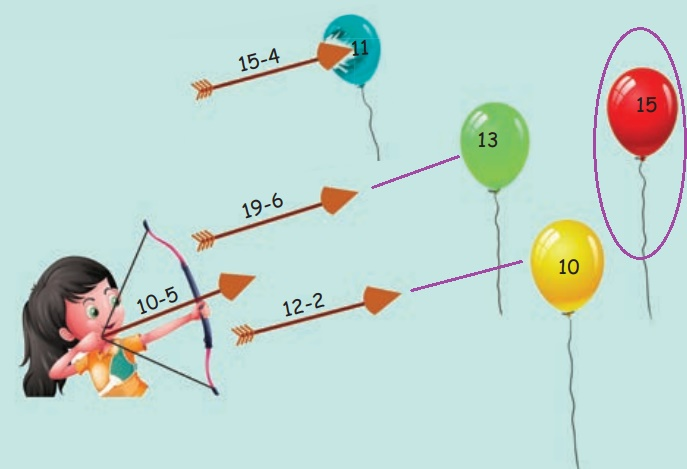Mental math (Oral)

• There are 18,drinking honey in a garden, After sometime 6 of them returned to their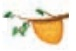. How manywill be left?

•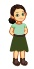saw 16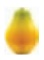in a tree. The next dayfound that 5fell down. How manyare remaining in the tree now?

• A parking area can accommodate 15. If 10were already parked in it then how many morecould be parked there?

Pleasure time

Complete the path to reach the victory cup.Numbers

Recall

How many tender coconuts are there in each box?Draw the correct number of dots in each box.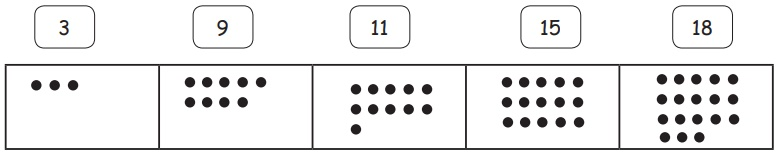Draw fewer and more for the given pictures. One is done for you.Teacher's Note

Teacher has to facilitate the children to draw fewer as well as more pictures in any possible ways with respect to the pictures given in the middle column.Write the missing numbers on the number line.What comes after the given number?

6 7

10 11

15 16

19 20

What comes before the given number?

2 3

7 8

12 13

16 17

What comes in between the given numbers?

4 5 6

12 13 14

17 18 19

Count and write the number of beads that you see. Draw one more bead and write the new total. One is done for you.Complete the table.Draw more beads to show the given number.Tags : Numbers | Term 3 Chapter 2 | 1st Maths , 1st Maths : Term 3 Unit 2 : Numbers
Study Material, Lecturing Notes, Assignment, Reference, Wiki description explanation, brief detail
1st Maths : Term 3 Unit 2 : Numbers : Subtraction (upto 20) | Numbers | Term 3 Chapter 2 | 1st Maths